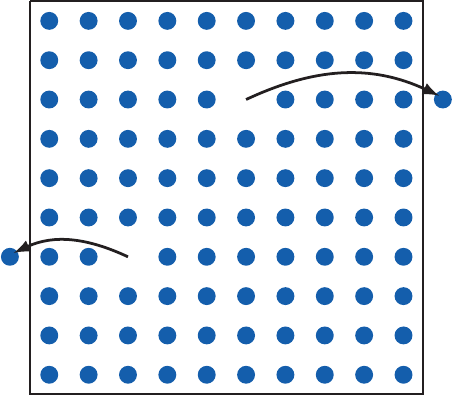Previous: A The Asymptotic Limit of the Phase Field Model    Top: Home    Next: Bibliography

### B Vacancies in a Crystal Lattice

According to [16, 51] the appearance of vacancies in a lattice can be explained by thermodynamical arguments. Suppose atoms are taken away from lattice positions and migrated to the surface leaving back vacancies. This kind of defects are called Schottky defects illustrated in Figure B.1. By assuming an atom positioned on a lattice site has the energyand an empty position has zero energy the state energy of a lattice withdefects can be expressed byFigure B.1.: Figure B.1.: Crystal lattice with atoms migrated to the surface leaving back Schottky defects .

As the vacancies are indistinguishable the number of distinct ways of distributingvacancies in a lattice withlattice sites is given byFor a micro-canonical assembly, the entropy is defined byEmploying Stirling’s approximation for bigand omitting the terms of the order ofresults inWith (B.1) the entropy can be expressed as a function of the state energy and can further be related to the temperature byresulting inThe final result can be rewritten givingwhich makes only sense when the ratio is much less then one. Otherwise the solid would by perforated with holes and collapse. For a typical energy valueofis in the range of 39 and the ratio of vacancies to the total available sites is in the order ofat room temperature. For small temperature the vacancy to lattice sites ratio function converges toand has the form of an Arrhenius law used in the modeling of electromigration introduced in Chapter 3.
The specific heat for vacancy formation can be calculated byand vanishes at low temperature due to the excitation gaps. At high temperature the energy spectrum is bounded from above. For practically used materials not only the configuration entropy but also other entropies like the vibration entropy are present.
Still the derivation shows that in a real crystal lattice vacancies are always present.

Previous: A The Asymptotic Limit of the Phase Field Model    Top: Home    Next: Bibliography